# class TF1: public TFormula, public TAttLine, public TAttFill, public TAttMarker

```
```

## TF1: 1-Dim function class

A TF1 object is a 1-Dim function defined between a lower and upper limit.
The function may be a simple function (see TFormula) or a precompiled user function.
The function may have associated parameters.
TF1 graphics function is via the TH1/TGraph drawing functions.

The following types of functions can be created:

### A - Expression using variable x and no parameters

#### Case 1: inline expression using standard C++ functions/operators

```   TF1 *fa1 = new TF1("fa1","sin(x)/x",0,10);
fa1->Draw();
```
```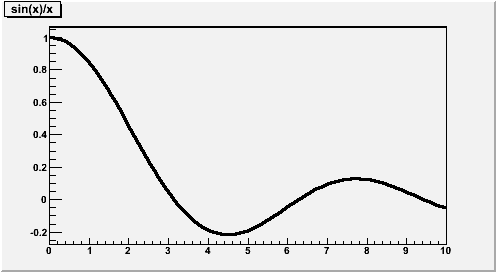```

#### Case 2: inline expression using TMath functions without parameters

```   TF1 *fa2 = new TF1("fa2","TMath::DiLog(x)",0,10);
fa2->Draw();
```
```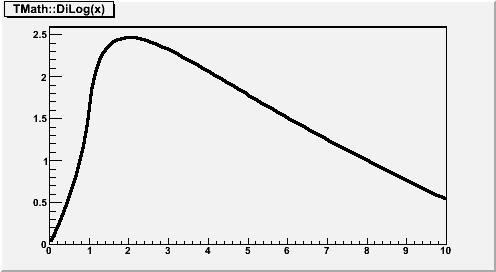```

#### Case 3: inline expression using a CINT function by name

```   Double_t myFunc(x) {
return x+sin(x);
}
TF1 *fa3 = new TF1("fa3","myFunc(x)",-3,5);
fa3->Draw();
```

### B - Expression using variable x with parameters

#### Case 1: inline expression using standard C++ functions/operators

• Example a:
> TF1 *fa = new TF1("fa","*x*sin(*x)",-3,3);
This creates a function of variable x with 2 parameters. The parameters must be initialized via:
```   fa->SetParameter(0,value_first_parameter);
fa->SetParameter(1,value_second_parameter);
```
Parameters may be given a name:
```   fa->SetParName(0,"Constant");
```
• Example b:
> TF1 *fb = new TF1("fb","gaus(0)*expo(3)",0,10);
gaus(0) is a substitute for *exp(-0.5*((x-)/)**2) and (0) means start numbering parameters at 0. expo(3) is a substitute for exp(+*x).

#### Case 2: inline expression using TMath functions with parameters

> TF1 *fb2 = new TF1("fa3","TMath::Landau(x,,,0)",-5,10); fb2->SetParameters(0.2,1.3); fb2->Draw();
```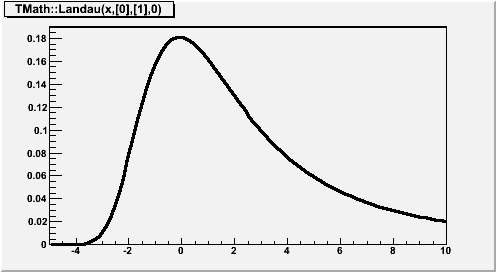```

### C - A general C function with parameters

Consider the macro myfunc.C below:
```   // Macro myfunc.C
Double_t myfunction(Double_t *x, Double_t *par)
{
Float_t xx =x;
Double_t f = TMath::Abs(par*sin(par*xx)/xx);
return f;
}
void myfunc()
{
TF1 *f1 = new TF1("myfunc",myfunction,0,10,2);
f1->SetParameters(2,1);
f1->SetParNames("constant","coefficient");
f1->Draw();
}
void myfit()
{
TH1F *h1=new TH1F("h1","test",100,0,10);
h1->FillRandom("myfunc",20000);
TF1 *f1=gROOT->GetFunction("myfunc");
f1->SetParameters(800,1);
h1.Fit("myfunc");
}
```

In an interactive session you can do:

```   Root > .L myfunc.C
Root > myfunc();
Root > myfit();
```
TF1 objects can reference other TF1 objects (thanks John
Odonnell) of type A or B defined above. This excludes CINT interpreted functions
and compiled functions. However, there is a restriction. A function cannot
reference a basic function if the basic function is a polynomial polN.

Example:

```   {
TF1 *fcos = new TF1 ("fcos", "*cos(x)", 0., 10.);
fcos->SetParNames( "cos");
fcos->SetParameter( 0, 1.1);
TF1 *fsin = new TF1 ("fsin", "*sin(x)", 0., 10.);
fsin->SetParNames( "sin");
fsin->SetParameter( 0, 2.1);
TF1 *fsincos = new TF1 ("fsc", "fcos+fsin");
TF1 *fs2 = new TF1 ("fs2", "fsc+fsc");
}
```

### D - A general C++ function object (functor) with parameters

A TF1 can be created from any C++ class implementing the operator()(double *x, double *p). The advantage of the function object is that he can have a state and reference therefore what-ever other object. In this way the user can customize his function.

Example:

```class  MyFunctionObject {
public:
// use constructor to customize your function object
double operator() (double *x, double *p) {
// function implementation using class data members
}
};
{
....
MyFunctionObject * fobj = new MyFunctionObject(....);       // create the function object
TF1 * f = new TF1("f",fobj,0,1,npar,"MyFunctionObject");    // create TF1 class.
.....
}
```
When constructing the TF1 class, the name of the function object class is required only if running in CINT and it is not needed in compiled C++ mode. In addition in compiled mode the cfnution object can be passed to TF1 by value. See also the tutorial math/exampleFunctor.C for a running example.

### E - A member function with parameters of a general C++ class

A TF1 can be created in this case from any member function of a class which has the signature of (double * , double *) and returning a double.

Example:

```class  MyFunction {
public:
...
double Evaluate() (double *x, double *p) {
// function implementation
}
};
{
....
MyFunction * fptr = new MyFunction(....);  // create the user function class
TF1 * f = new TF1("f",fptr,&MyFunction::Evaluate,0,1,npar,"MyFunction","Evaluate");   // create TF1 class.
.....
}
```
When constructing the TF1 class, the name of the function class and of the member function are required only if running in CINT and they are not need in compiled C++ mode. See also the tutorial math/exampleFunctor.C for a running example.
```
```

## Function Members (Methods)

public:
 TF1() TF1(const TF1& f1) TF1(const char* name, const char* formula, Double_t xmin = 0, Double_t xmax = 1) TF1(const char* name, Double_t xmin, Double_t xmax, Int_t npar) TF1(const char* name, void* fcn, Double_t xmin, Double_t xmax, Int_t npar) TF1(const char* name, ROOT::Math::ParamFunctor f, Double_t xmin = 0, Double_t xmax = 1, Int_t npar = 0) TF1(const char* name, void* ptr, Double_t xmin, Double_t xmax, Int_t npar, char* className) TF1(const char* name, void* ptr, void*, Double_t xmin, Double_t xmax, Int_t npar, char* className, char* methodName = 0) virtual ~TF1() void TObject::AbstractMethod(const char* method) const static void AbsValue(Bool_t reject = kTRUE) virtual void TFormula::Analyze(const char* schain, Int_t& err, Int_t offset = 0) virtual Bool_t TFormula::AnalyzeFunction(TString& chaine, Int_t& err, Int_t offset = 0) virtual void TObject::AppendPad(Option_t* option = "") virtual void Browse(TBrowser* b) static void CalcGaussLegendreSamplingPoints(Int_t num, Double_t* x, Double_t* w, Double_t eps = 3.0e-11) virtual Double_t CentralMoment(Double_t n, Double_t a, Double_t b, const Double_t* params = 0, Double_t epsilon = 0.000001) static TClass* Class() virtual const char* TObject::ClassName() const virtual void TFormula::Clear(Option_t* option = "") virtual TObject* TNamed::Clone(const char* newname = "") const virtual Int_t TNamed::Compare(const TObject* obj) const virtual Int_t TFormula::Compile(const char* expression = "") virtual void Copy(TObject& f1) const virtual char* TFormula::DefinedString(Int_t code) virtual Double_t TFormula::DefinedValue(Int_t code) virtual Int_t TFormula::DefinedVariable(TString& variable, Int_t& action) virtual void TObject::Delete(Option_t* option = "") virtual Double_t Derivative(Double_t x, Double_t* params = 0, Double_t epsilon = 0.001) const virtual Double_t Derivative2(Double_t x, Double_t* params = 0, Double_t epsilon = 0.001) const virtual Double_t Derivative3(Double_t x, Double_t* params = 0, Double_t epsilon = 0.001) const static Double_t DerivativeError() Int_t TAttLine::DistancetoLine(Int_t px, Int_t py, Double_t xp1, Double_t yp1, Double_t xp2, Double_t yp2) virtual Int_t DistancetoPrimitive(Int_t px, Int_t py) virtual void Draw(Option_t* option = "") virtual void TObject::DrawClass() const virtual TObject* TObject::DrawClone(Option_t* option = "") const virtual TF1* DrawCopy(Option_t* option = "") const virtual void DrawDerivative(Option_t* option = "al") virtual void DrawF1(const char* formula, Double_t xmin, Double_t xmax, Option_t* option = "") virtual void DrawIntegral(Option_t* option = "al") virtual void TObject::Dump() const virtual void TObject::Error(const char* method, const char* msgfmt) const virtual Double_t Eval(Double_t x, Double_t y = 0, Double_t z = 0, Double_t t = 0) const virtual Double_t EvalPar(const Double_t* x, const Double_t* params = 0) virtual Double_t TFormula::EvalParOld(const Double_t* x, const Double_t* params = 0) virtual void TObject::Execute(const char* method, const char* params, Int_t* error = 0) virtual void TObject::Execute(TMethod* method, TObjArray* params, Int_t* error = 0) virtual void ExecuteEvent(Int_t event, Int_t px, Int_t py) virtual void TObject::Fatal(const char* method, const char* msgfmt) const virtual void TNamed::FillBuffer(char*& buffer) virtual TObject* TObject::FindObject(const char* name) const virtual TObject* TObject::FindObject(const TObject* obj) const virtual void FixParameter(Int_t ipar, Double_t value) Double_t GetChisquare() const static TF1* GetCurrent() virtual Option_t* TObject::GetDrawOption() const static Long_t TObject::GetDtorOnly() virtual TString TFormula::GetExpFormula(Option_t* option = "") const virtual Color_t TAttFill::GetFillColor() const virtual Style_t TAttFill::GetFillStyle() const TH1* GetHistogram() const virtual const char* TObject::GetIconName() const virtual const TObject* TFormula::GetLinearPart(Int_t i) virtual Color_t TAttLine::GetLineColor() const virtual Style_t TAttLine::GetLineStyle() const virtual Width_t TAttLine::GetLineWidth() const virtual Color_t TAttMarker::GetMarkerColor() const virtual Size_t TAttMarker::GetMarkerSize() const virtual Style_t TAttMarker::GetMarkerStyle() const virtual Double_t GetMaximum(Double_t xmin = 0, Double_t xmax = 0) const virtual Double_t GetMaximumX(Double_t xmin = 0, Double_t xmax = 0) const TMethodCall* GetMethodCall() const virtual Double_t GetMinimum(Double_t xmin = 0, Double_t xmax = 0) const virtual Double_t GetMinimumX(Double_t xmin = 0, Double_t xmax = 0) const virtual const char* TNamed::GetName() const virtual Int_t GetNDF() const virtual Int_t TFormula::GetNdim() const virtual Int_t TFormula::GetNpar() const virtual Int_t GetNpx() const virtual Int_t TFormula::GetNumber() const virtual Int_t GetNumberFitPoints() const virtual Int_t GetNumberFreeParameters() const virtual char* GetObjectInfo(Int_t px, Int_t py) const static Bool_t TObject::GetObjectStat() virtual Option_t* TObject::GetOption() const Double_t TFormula::GetParameter(Int_t ipar) const Double_t TFormula::GetParameter(const char* name) const virtual Double_t* TFormula::GetParameters() const virtual void TFormula::GetParameters(Double_t* params) TObject* GetParent() const virtual Double_t GetParError(Int_t ipar) const virtual Double_t* GetParErrors() const virtual void GetParLimits(Int_t ipar, Double_t& parmin, Double_t& parmax) const virtual const char* TFormula::GetParName(Int_t ipar) const virtual Int_t TFormula::GetParNumber(const char* name) const virtual Double_t GetProb() const virtual Int_t GetQuantiles(Int_t nprobSum, Double_t* q, const Double_t* probSum) virtual Double_t GetRandom() virtual Double_t GetRandom(Double_t xmin, Double_t xmax) virtual void GetRange(Double_t& xmin, Double_t& xmax) const virtual void GetRange(Double_t& xmin, Double_t& ymin, Double_t& xmax, Double_t& ymax) const virtual void GetRange(Double_t& xmin, Double_t& ymin, Double_t& zmin, Double_t& xmax, Double_t& ymax, Double_t& zmax) const virtual Double_t GetSave(const Double_t* x) virtual const char* TNamed::GetTitle() const virtual UInt_t TObject::GetUniqueID() const virtual Double_t GetX(Double_t y, Double_t xmin = 0, Double_t xmax = 0) const TAxis* GetXaxis() const virtual Double_t GetXmax() const virtual Double_t GetXmin() const TAxis* GetYaxis() const TAxis* GetZaxis() const virtual Double_t GradientPar(Int_t ipar, const Double_t* x, Double_t eps = 0.01) virtual void GradientPar(const Double_t* x, Double_t* grad, Double_t eps = 0.01) virtual Bool_t TObject::HandleTimer(TTimer* timer) virtual ULong_t TNamed::Hash() const virtual void TObject::Info(const char* method, const char* msgfmt) const virtual Bool_t TObject::InheritsFrom(const char* classname) const virtual Bool_t TObject::InheritsFrom(const TClass* cl) const virtual void InitArgs(const Double_t* x, const Double_t* params) static void InitStandardFunctions() virtual void TObject::Inspect() const virtual Double_t Integral(Double_t a, Double_t b, const Double_t* params = 0, Double_t epsilon = 1e-12) virtual Double_t Integral(Double_t ax, Double_t bx, Double_t ay, Double_t by, Double_t epsilon = 1e-12) virtual Double_t Integral(Double_t ax, Double_t bx, Double_t ay, Double_t by, Double_t az, Double_t bz, Double_t epsilon = 1e-12) virtual Double_t IntegralError(Double_t a, Double_t b, Double_t epsilon = 1e-12) virtual Double_t IntegralFast(Int_t num, Double_t* x, Double_t* w, Double_t a, Double_t b, Double_t* params = 0) virtual Double_t IntegralMultiple(Int_t n, const Double_t* a, const Double_t* b, Double_t epsilon, Double_t& relerr) virtual Double_t IntegralMultiple(Int_t n, const Double_t* a, const Double_t* b, Int_t minpts, Int_t maxpts, Double_t epsilon, Double_t& relerr, Int_t& nfnevl, Int_t& ifail) void TObject::InvertBit(UInt_t f) virtual TClass* IsA() const virtual Bool_t TObject::IsEqual(const TObject* obj) const virtual Bool_t TObject::IsFolder() const virtual Bool_t IsInside(const Double_t* x) const virtual Bool_t TFormula::IsLinear() virtual Bool_t TFormula::IsNormalized() Bool_t TObject::IsOnHeap() const virtual Bool_t TNamed::IsSortable() const virtual Bool_t TAttFill::IsTransparent() const Bool_t TObject::IsZombie() const virtual void TNamed::ls(Option_t* option = "") const void TObject::MayNotUse(const char* method) const virtual Double_t Mean(Double_t a, Double_t b, const Double_t* params = 0, Double_t epsilon = 0.000001) Double_t MinimBrent(Int_t type, Double_t& xmin, Double_t& xmax, Double_t xmiddle, Double_t fy, Bool_t& ok) const Double_t MinimStep(Int_t type, Double_t& xmin, Double_t& xmax, Double_t fy) const virtual void TAttLine::Modify() virtual Double_t Moment(Double_t n, Double_t a, Double_t b, const Double_t* params = 0, Double_t epsilon = 0.000001) virtual Bool_t TObject::Notify() static void TObject::operator delete(void* ptr) static void TObject::operator delete(void* ptr, void* vp) static void TObject::operator delete[](void* ptr) static void TObject::operator delete[](void* ptr, void* vp) void* TObject::operator new(size_t sz) void* TObject::operator new(size_t sz, void* vp) void* TObject::operator new[](size_t sz) void* TObject::operator new[](size_t sz, void* vp) virtual Double_t operator()(const Double_t* x, const Double_t* params = 0) TF1& operator=(const TF1& rhs) void TFormula::Optimize() virtual void Paint(Option_t* option = "") virtual void TObject::Pop() virtual void Print(Option_t* option = "") const virtual void TFormula::ProcessLinear(TString& replaceformula) virtual Int_t TObject::Read(const char* name) virtual void TObject::RecursiveRemove(TObject* obj) static Bool_t RejectedPoint() static void RejectPoint(Bool_t reject = kTRUE) virtual void ReleaseParameter(Int_t ipar) virtual void TAttFill::ResetAttFill(Option_t* option = "") virtual void TAttLine::ResetAttLine(Option_t* option = "") virtual void TAttMarker::ResetAttMarker(Option_t* toption = "") void TObject::ResetBit(UInt_t f) virtual void Save(Double_t xmin, Double_t xmax, Double_t ymin, Double_t ymax, Double_t zmin, Double_t zmax) virtual void TObject::SaveAs(const char* filename = "", Option_t* option = "") const virtual void TAttFill::SaveFillAttributes(ostream& out, const char* name, Int_t coldef = 1, Int_t stydef = 1001) virtual void TAttLine::SaveLineAttributes(ostream& out, const char* name, Int_t coldef = 1, Int_t stydef = 1, Int_t widdef = 1) virtual void TAttMarker::SaveMarkerAttributes(ostream& out, const char* name, Int_t coldef = 1, Int_t stydef = 1, Int_t sizdef = 1) virtual void SavePrimitive(ostream& out, Option_t* option = "") void TObject::SetBit(UInt_t f) void TObject::SetBit(UInt_t f, Bool_t set) virtual void SetChisquare(Double_t chi2) static void SetCurrent(TF1* f1) virtual void TObject::SetDrawOption(Option_t* option = "") static void TObject::SetDtorOnly(void* obj) virtual void TAttFill::SetFillAttributes() virtual void TAttFill::SetFillColor(Color_t fcolor) virtual void TAttFill::SetFillStyle(Style_t fstyle) virtual void SetFunction(Double_t (*)(Double_t*, Double_t*) fcn) virtual void TAttLine::SetLineAttributes() virtual void TAttLine::SetLineColor(Color_t lcolor) virtual void TAttLine::SetLineStyle(Style_t lstyle) virtual void TAttLine::SetLineWidth(Width_t lwidth) virtual void TAttMarker::SetMarkerAttributes() virtual void TAttMarker::SetMarkerColor(Color_t tcolor = 1) virtual void TAttMarker::SetMarkerSize(Size_t msize = 1) virtual void TAttMarker::SetMarkerStyle(Style_t mstyle = 1) static void TFormula::SetMaxima(Int_t maxop = 1000, Int_t maxpar = 1000, Int_t maxconst = 1000) virtual void SetMaximum(Double_t maximum = -1111) virtual void SetMinimum(Double_t minimum = -1111) virtual void TNamed::SetName(const char* name) virtual void TNamed::SetNameTitle(const char* name, const char* title) virtual void SetNDF(Int_t ndf) virtual void SetNpx(Int_t npx = 100) virtual void TFormula::SetNumber(Int_t number) virtual void SetNumberFitPoints(Int_t npfits) static void TObject::SetObjectStat(Bool_t stat) virtual void TFormula::SetParameter(const char* name, Double_t parvalue) virtual void TFormula::SetParameter(Int_t ipar, Double_t parvalue) virtual void TFormula::SetParameters(const Double_t* params) virtual void TFormula::SetParameters(Double_t p0, Double_t p1, Double_t p2 = 0, Double_t p3 = 0, Double_t p4 = 0, Double_t p5 = 0, Double_t p6 = 0, Double_t p7 = 0, Double_t p8 = 0, Double_t p9 = 0, Double_t p10 = 0) virtual void SetParent(TObject* p = 0) virtual void SetParError(Int_t ipar, Double_t error) virtual void SetParErrors(const Double_t* errors) virtual void SetParLimits(Int_t ipar, Double_t parmin, Double_t parmax) virtual void TFormula::SetParName(Int_t ipar, const char* name) virtual void TFormula::SetParNames(const char* name0 = "p0", const char* name1 = "p1", const char* name2 = "p2", const char* name3 = "p3", const char* name4 = "p4", const char* name5 = "p5", const char* name6 = "p6", const char* name7 = "p7", const char* name8 = "p8", const char* name9 = "p9", const char* name10 = "p10") virtual void SetRange(Double_t xmin, Double_t xmax) virtual void SetRange(Double_t xmin, Double_t ymin, Double_t xmax, Double_t ymax) virtual void SetRange(Double_t xmin, Double_t ymin, Double_t zmin, Double_t xmax, Double_t ymax, Double_t zmax) virtual void SetSavedPoint(Int_t point, Double_t value) virtual void SetTitle(const char* title = "") virtual void TObject::SetUniqueID(UInt_t uid) virtual void ShowMembers(TMemberInspector& insp, char* parent) virtual Int_t TNamed::Sizeof() const virtual void Streamer(TBuffer& b) void StreamerNVirtual(TBuffer& b) virtual void TObject::SysError(const char* method, const char* msgfmt) const Bool_t TObject::TestBit(UInt_t f) const Int_t TObject::TestBits(UInt_t f) const virtual void Update() virtual void TObject::UseCurrentStyle() virtual Double_t Variance(Double_t a, Double_t b, const Double_t* params = 0, Double_t epsilon = 0.000001) virtual void TObject::Warning(const char* method, const char* msgfmt) const virtual Int_t TObject::Write(const char* name = 0, Int_t option = 0, Int_t bufsize = 0) virtual Int_t TObject::Write(const char* name = 0, Int_t option = 0, Int_t bufsize = 0) const
protected:
 void TFormula::ClearFormula(Option_t* option = "") virtual void TFormula::Convert(UInt_t fromVersion) void CreateFromCintClass(const char* name, void* ptr, Double_t xmin, Double_t xmax, Int_t npar, char* cname, char* fname) void CreateFromFunctor(const char* name, Int_t npar) virtual void TObject::DoError(int level, const char* location, const char* fmt, va_list va) const Double_t TFormula::EvalParFast(const Double_t* x, const Double_t* params) Double_t TFormula::EvalPrimitive(const Double_t* x, const Double_t* params) Double_t TFormula::EvalPrimitive0(const Double_t* x, const Double_t* params) Double_t TFormula::EvalPrimitive1(const Double_t* x, const Double_t* params) Double_t TFormula::EvalPrimitive2(const Double_t* x, const Double_t* params) Double_t TFormula::EvalPrimitive3(const Double_t* x, const Double_t* params) Double_t TFormula::EvalPrimitive4(const Double_t* x, const Double_t* params) Short_t TFormula::GetAction(Int_t code) const Short_t TFormula::GetActionOptimized(Int_t code) const Int_t TFormula::GetActionParam(Int_t code) const Int_t TFormula::GetActionParamOptimized(Int_t code) const Int_t* TFormula::GetOper() const Int_t* TFormula::GetOperOptimized() const virtual Bool_t TFormula::IsString(Int_t oper) const void TFormula::MakePrimitive(const char* expr, Int_t pos) void TObject::MakeZombie() Int_t TFormula::PreCompile() void TFormula::SetAction(Int_t code, Int_t value, Int_t param = 0) void TFormula::SetActionOptimized(Int_t code, Int_t value, Int_t param = 0)

## Data Members

public:
 enum { kNotDraw }; enum TFormula::[unnamed] { kEnd kAdd kSubstract kMultiply kDivide kModulo kcos ksin ktan kacos kasin katan katan2 kfmod kpow ksq ksqrt kstrstr kmin kmax klog kexp klog10 kpi kabs ksign kint kSignInv krndm kAnd kOr kEqual kNotEqual kLess kGreater kLessThan kGreaterThan kNot kcosh ksinh ktanh kacosh kasinh katanh kStringEqual kStringNotEqual kBitAnd kBitOr kLeftShift kRightShift kexpo kxexpo kyexpo kzexpo kxyexpo kgaus kxgaus kygaus kzgaus kxygaus klandau kxlandau kylandau kzlandau kxylandau kpol kxpol kypol kzpol kParameter kConstant kBoolOptimize kStringConst kVariable kFunctionCall kData kUnary kBinary kThree kDefinedVariable kDefinedString kPlusD kPlusDD kMultD kMultDD kBoolOptimizeOr kBoolOptimizeAnd kBoolSet kFDM kFD0 kFD1 kFD2 kFD3 kNotGlobal kNormalized kLinear }; enum TObject::EStatusBits { kCanDelete kMustCleanup kObjInCanvas kIsReferenced kHasUUID kCannotPick kNoContextMenu kInvalidObject }; enum TObject::[unnamed] { kIsOnHeap kNotDeleted kZombie kBitMask kSingleKey kOverwrite kWriteDelete };
public:
 G__p2memfunc TFormula::fOptimal !pointer to optimal function
protected:
 Double_t* fAlpha !Array alpha. for each bin in x the deconvolution r of fIntegral TBits TFormula::fAlreadyFound ! cache for information Double_t* fBeta !Array beta. is approximated by x = alpha +beta*r *gamma*r**2 Double_t fChisquare Function fit chisquare void* fCintFunc ! pointer to interpreted function class Double_t* TFormula::fConst [fNconst] Array of fNconst formula constants TString* TFormula::fExpr [fNoper] List of expressions TString* TFormula::fExprOptimized ![fNOperOptimized] List of expressions Color_t TAttFill::fFillColor fill area color Style_t TAttFill::fFillStyle fill area style TObjArray TFormula::fFunctions Array of function calls to make ROOT::Math::ParamFunctor fFunctor ! Functor object to wrap any C++ callable object Double_t* fGamma !Array gamma. TH1* fHistogram !Pointer to histogram used for visualisation Double_t* fIntegral ![fNpx] Integral of function binned on fNpx bins Color_t TAttLine::fLineColor line color Style_t TAttLine::fLineStyle line style Width_t TAttLine::fLineWidth line width TObjArray TFormula::fLinearParts ! Linear parts if the formula is linear (contains '|') Color_t TAttMarker::fMarkerColor Marker color index Size_t TAttMarker::fMarkerSize Marker size Style_t TAttMarker::fMarkerStyle Marker style Double_t fMaximum Maximum value for plotting TMethodCall* fMethodCall !Pointer to MethodCall in case of interpreted function Double_t fMinimum Minimum value for plotting Int_t fNDF Number of degrees of freedom in the fit Int_t TFormula::fNOperOptimized !Number of operators after optimization TString TNamed::fName object identifier TString* TFormula::fNames [fNpar] Array of parameter names Int_t TFormula::fNconst Number of constants Int_t TFormula::fNdim Dimension of function (1=1-Dim, 2=2-Dim,etc) Int_t TFormula::fNoper Number of operators Int_t TFormula::fNpar Number of parameters Int_t fNpfits Number of points used in the fit Int_t fNpx Number of points used for the graphical representation Int_t fNsave Number of points used to fill array fSave Int_t TFormula::fNstring Number of different constants character strings Int_t TFormula::fNumber formula number identifier Int_t TFormula::fNval Number of different variables in expression TOperOffset* TFormula::fOperOffset ![fNOperOptimized] Offsets of operrands Int_t* TFormula::fOperOptimized ![fNOperOptimized] List of operators. (See documentation for changes made at version 7) Double_t* fParErrors [fNpar] Array of errors of the fNpar parameters Double_t* fParMax [fNpar] Array of upper limits of the fNpar parameters Double_t* fParMin [fNpar] Array of lower limits of the fNpar parameters Double_t* TFormula::fParams [fNpar] Array of fNpar parameters TObject* fParent !Parent object hooking this function (if one) TFormulaPrimitive** TFormula::fPredefined ![fNPar] predefined function Double_t* fSave [fNsave] Array of fNsave function values TString TNamed::fTitle object title Int_t fType (=0 for standard functions, 1 if pointer to function) Double_t fXmax Upper bounds for the range Double_t fXmin Lower bounds for the range static Bool_t fgAbsValue use absolute value of function when computing integral static TF1* fgCurrent pointer to current function being processed static Bool_t fgRejectPoint True if point must be rejected in a fit

## Class Charts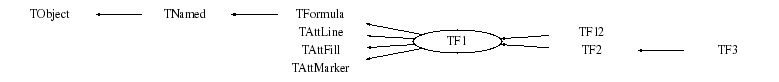## Function documentation

TF1(const TF1& f1)
``` F1 default constructor.
```
TF1(const char *name,const char *formula, Double_t xmin, Double_t xmax)
``` F1 constructor using a formula definition

See TFormula constructor for explanation of the formula syntax.

See tutorials: fillrandom, first, fit1, formula1, multifit
for real examples.

Creates a function of type A or B between xmin and xmax

if formula has the form "fffffff;xxxx;yyyy", it is assumed that
the formula string is "fffffff" and "xxxx" and "yyyy" are the
titles for the X and Y axis respectively.
```
TF1(const char *name, Double_t xmin, Double_t xmax, Int_t npar)
``` F1 constructor using name of an interpreted function.

Creates a function of type C between xmin and xmax.
name is the name of an interpreted CINT cunction.
The function is defined with npar parameters
fcn must be a function of type:
Double_t fcn(Double_t *x, Double_t *params)

This constructor is called for functions of type C by CINT.

WARNING! A function created with this constructor cannot be Cloned.
```
TF1(const char *name,void *fcn, Double_t xmin, Double_t xmax, Int_t npar)
``` F1 constructor using pointer to an interpreted function.

See TFormula constructor for explanation of the formula syntax.

Creates a function of type C between xmin and xmax.
The function is defined with npar parameters
fcn must be a function of type:
Double_t fcn(Double_t *x, Double_t *params)

see tutorial; myfit for an example of use
also test/stress.cxx (see function stress1)

This constructor is called for functions of type C by CINT.

WARNING! A function created with this constructor cannot be Cloned.
```
TF1(const char* name, void* ptr, Double_t xmin, Double_t xmax, Int_t npar, char* className)
``` F1 constructor using a pointer to a real function.

npar is the number of free parameters used by the function

This constructor creates a function of type C when invoked
with the normal C++ compiler.

see test program test/stress.cxx (function stress1) for an example.
note the interface with an intermediate pointer.

WARNING! A function created with this constructor cannot be Cloned.
```
TF1(const char* name, void* ptr, Double_t xmin, Double_t xmax, Int_t npar, char* className)
``` F1 constructor using a pointer to real function.

npar is the number of free parameters used by the function

This constructor creates a function of type C when invoked
with the normal C++ compiler.

see test program test/stress.cxx (function stress1) for an example.
note the interface with an intermediate pointer.

WARNING! A function created with this constructor cannot be Cloned.
```
TF1(const char *name, ROOT::Math::ParamFunctor f, Double_t xmin, Double_t xmax, Int_t npar )
``` F1 constructor using the Functor class.

xmin and xmax define the plotting range of the function
npar is the number of free parameters used by the function

This constructor can be used only in compiled code

WARNING! A function created with this constructor cannot be Cloned.
```
void CreateFromFunctor(const char* name, Int_t npar)
``` Internal Function to Create a TF1  using a Functor.

Used by the template constructors
```
TF1(const char* name, void* ptr, Double_t xmin, Double_t xmax, Int_t npar, char* className)
``` F1 constructor from an interpreted class defining the operator() or Eval().
This constructor emulate the syntax of the template constructor using a C++ callable object (functor)
which can be used only in C++ compiled mode.
The class name is required to get the type of class given the void pointer ptr.
For the method name is used the operator() (double *, double * ).
Use the other constructor taking the method name for different method names.

xmin and xmax specify the function plotting range
npar are the number of function parameters

see tutorial  math.exampleFunctor.C for an example of using this constructor

This constructor is used only when using CINT.
In compiled mode the template constructor is used and in that case className is not needed
```
void CreateFromCintClass(const char* name, void* ptr, Double_t xmin, Double_t xmax, Int_t npar, char* cname, char* fname)
``` Internal function used to create from TF1 from an interpreter CINT class
with the specified type (className) and member function name (methodName).

```
TF1& operator=(const TF1& rhs)
``` Operator =
```
~TF1()
``` TF1 default destructor.
```
void AbsValue(Bool_t reject = kTRUE)
``` Static function: set the fgAbsValue flag.
By default TF1::Integral uses the original function value to compute the integral
However, TF1::Moment, CentralMoment require to compute the integral
using the absolute value of the function.
```
void Browse(TBrowser* b)
``` Browse.
```
void Copy(TObject& f1) const
``` Copy this F1 to a new F1.
```
Double_t Derivative(Double_t x, Double_t* params = 0, Double_t epsilon = 0.001) const
``` Returns the first derivative of the function at point x,
computed by Richardson's extrapolation method (use 2 derivative estimates
to compute a third, more accurate estimation)
first, derivatives with steps h and h/2 are computed by central difference formulas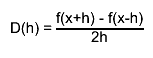$D(h) = #frac{f(x+h) - f(x-h)}{2h}$
the final estimate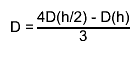$D = #frac{4D(h/2) - D(h)}{3}$
"Numerical Methods for Scientists and Engineers", H.M.Antia, 2nd edition"

if the argument params is null, the current function parameters are used,
otherwise the parameters in params are used.

the argument eps may be specified to control the step size (precision).
the step size is taken as eps*(xmax-xmin).
the default value (0.001) should be good enough for the vast majority
of functions. Give a smaller value if your function has many changes
of the second derivative in the function range.

Getting the error via TF1::DerivativeError:
(total error = roundoff error + interpolation error)
the estimate of the roundoff error is taken as follows: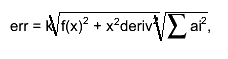$err = k#sqrt{f(x)^{2} + x^{2}deriv^{2}}#sqrt{#sum ai^{2}},$
where k is the double precision, ai are coefficients used in
central difference formulas
interpolation error is decreased by making the step size h smaller.

Author: Anna Kreshuk
```
Double_t Derivative2(Double_t x, Double_t* params = 0, Double_t epsilon = 0.001) const
``` Returns the second derivative of the function at point x,
computed by Richardson's extrapolation method (use 2 derivative estimates
to compute a third, more accurate estimation)
first, derivatives with steps h and h/2 are computed by central difference formulas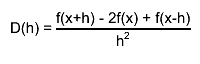$D(h) = #frac{f(x+h) - 2f(x) + f(x-h)}{h^{2}}$
the final estimate$D = #frac{4D(h/2) - D(h)}{3}$
"Numerical Methods for Scientists and Engineers", H.M.Antia, 2nd edition"

if the argument params is null, the current function parameters are used,
otherwise the parameters in params are used.

the argument eps may be specified to control the step size (precision).
the step size is taken as eps*(xmax-xmin).
the default value (0.001) should be good enough for the vast majority
of functions. Give a smaller value if your function has many changes
of the second derivative in the function range.

Getting the error via TF1::DerivativeError:
(total error = roundoff error + interpolation error)
the estimate of the roundoff error is taken as follows:$err = k#sqrt{f(x)^{2} + x^{2}deriv^{2}}#sqrt{#sum ai^{2}},$
where k is the double precision, ai are coefficients used in
central difference formulas
interpolation error is decreased by making the step size h smaller.

Author: Anna Kreshuk
```
Double_t Derivative3(Double_t x, Double_t* params = 0, Double_t epsilon = 0.001) const
``` Returns the third derivative of the function at point x,
computed by Richardson's extrapolation method (use 2 derivative estimates
to compute a third, more accurate estimation)
first, derivatives with steps h and h/2 are computed by central difference formulas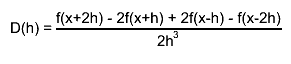$D(h) = #frac{f(x+2h) - 2f(x+h) + 2f(x-h) - f(x-2h)}{2h^{3}}$
the final estimate$D = #frac{4D(h/2) - D(h)}{3}$
"Numerical Methods for Scientists and Engineers", H.M.Antia, 2nd edition"

if the argument params is null, the current function parameters are used,
otherwise the parameters in params are used.

the argument eps may be specified to control the step size (precision).
the step size is taken as eps*(xmax-xmin).
the default value (0.001) should be good enough for the vast majority
of functions. Give a smaller value if your function has many changes
of the second derivative in the function range.

Getting the error via TF1::DerivativeError:
(total error = roundoff error + interpolation error)
the estimate of the roundoff error is taken as follows:$err = k#sqrt{f(x)^{2} + x^{2}deriv^{2}}#sqrt{#sum ai^{2}},$
where k is the double precision, ai are coefficients used in
central difference formulas
interpolation error is decreased by making the step size h smaller.

Author: Anna Kreshuk
```

``` Static function returning the error of the last call to the Derivative
functions
```

``` Compute distance from point px,py to a function.

Compute the closest distance of approach from point px,py to this
function. The distance is computed in pixels units.

Note that px is called with a negative value when the TF1 is in
TGraph or TH1 list of functions. In this case there is no point
looking at the histogram axis.
```
void Draw(Option_t* option = "")
``` Draw this function with its current attributes.

Possible option values are:
"SAME"  superimpose on top of existing picture
"L"     connect all computed points with a straight line
"C"     connect all computed points with a smooth curve.
"FC"    draw a fill area below a smooth curve

Note that the default value is "L". Therefore to draw on top
of an existing picture, specify option "LSAME"

NB. You must use DrawCopy if you want to draw several times the same
function in the current canvas.
```
TF1 * DrawCopy(Option_t* option = "") const
``` Draw a copy of this function with its current attributes.

This function MUST be used instead of Draw when you want to draw
the same function with different parameters settings in the same canvas.

Possible option values are:
"SAME"  superimpose on top of existing picture
"L"     connect all computed points with a straight line
"C"     connect all computed points with a smooth curve.
"FC"    draw a fill area below a smooth curve

Note that the default value is "L". Therefore to draw on top
of an existing picture, specify option "LSAME"
```
void DrawDerivative(Option_t* option = "al")
``` Draw derivative of this function

An intermediate TGraph object is built and drawn with option.

The resulting graph will be drawn into the current pad.
If this function is used via the context menu, it recommended
to create a new canvas/pad before invoking this function.
```
void DrawIntegral(Option_t* option = "al")
``` Draw integral of this function

An intermediate TGraph object is built and drawn with option.

The resulting graph will be drawn into the current pad.
If this function is used via the context menu, it recommended
to create a new canvas/pad before invoking this function.
```
void DrawF1(const char* formula, Double_t xmin, Double_t xmax, Option_t* option = "")
``` Draw formula between xmin and xmax.
```
Double_t Eval(Double_t x, Double_t y = 0, Double_t z = 0, Double_t t = 0) const
``` Evaluate this formula.

Computes the value of this function (general case for a 3-d function)
at point x,y,z.
For a 1-d function give y=0 and z=0
The current value of variables x,y,z is passed through x, y and z.
The parameters used will be the ones in the array params if params is given
otherwise parameters will be taken from the stored data members fParams
```
Double_t EvalPar(const Double_t* x, const Double_t* params = 0)
``` Evaluate function with given coordinates and parameters.

Compute the value of this function at point defined by array x
and current values of parameters in array params.
If argument params is omitted or equal 0, the internal values
of parameters (array fParams) will be used instead.
For a 1-D function only x must be given.
In case of a multi-dimemsional function, the arrays x must be
filled with the corresponding number of dimensions.

WARNING. In case of an interpreted function (fType=2), it is the
user's responsability to initialize the parameters via InitArgs
before calling this function.
InitArgs should be called at least once to specify the addresses
of the arguments x and params.
InitArgs should be called everytime these addresses change.
```
void ExecuteEvent(Int_t event, Int_t px, Int_t py)
``` Execute action corresponding to one event.

This member function is called when a F1 is clicked with the locator
```
void FixParameter(Int_t ipar, Double_t value)
``` Fix the value of a parameter
The specified value will be used in a fit operation
```

``` Static function returning the current function being processed
```

``` Return a pointer to the histogram used to vusualize the function
```
Double_t GetMaximum(Double_t xmin = 0, Double_t xmax = 0) const
``` Return the maximum value of the function
Method:
First, the grid search is used to bracket the maximum
with the step size = (xmax-xmin)/fNpx.
This way, the step size can be controlled via the SetNpx() function.
If the function is unimodal or if its extrema are far apart, setting
the fNpx to a small value speeds the algorithm up many times.
Then, Brent's method is applied on the bracketed interval
```
Double_t GetMaximumX(Double_t xmin = 0, Double_t xmax = 0) const
``` Return the X value corresponding to the maximum value of the function
Method:
First, the grid search is used to bracket the maximum
with the step size = (xmax-xmin)/fNpx.
This way, the step size can be controlled via the SetNpx() function.
If the function is unimodal or if its extrema are far apart, setting
the fNpx to a small value speeds the algorithm up many times.
Then, Brent's method is applied on the bracketed interval
```
Double_t GetMinimum(Double_t xmin = 0, Double_t xmax = 0) const
``` Returns the minimum value of the function on the (xmin, xmax) interval
Method:
First, the grid search is used to bracket the maximum
with the step size = (xmax-xmin)/fNpx. This way, the step size
can be controlled via the SetNpx() function. If the function is
unimodal or if its extrema are far apart, setting the fNpx to
a small value speeds the algorithm up many times.
Then, Brent's method is applied on the bracketed interval
```
Double_t GetMinimumX(Double_t xmin = 0, Double_t xmax = 0) const
``` Returns the X value corresponding to the minimum value of the function
on the (xmin, xmax) interval
Method:
First, the grid search is used to bracket the maximum
with the step size = (xmax-xmin)/fNpx. This way, the step size
can be controlled via the SetNpx() function. If the function is
unimodal or if its extrema are far apart, setting the fNpx to
a small value speeds the algorithm up many times.
Then, Brent's method is applied on the bracketed interval
```
Double_t GetX(Double_t y, Double_t xmin = 0, Double_t xmax = 0) const
``` Returns the X value corresponding to the function value fy for (xmin<x<xmax).
Method:
First, the grid search is used to bracket the maximum
with the step size = (xmax-xmin)/fNpx. This way, the step size
can be controlled via the SetNpx() function. If the function is
unimodal or if its extrema are far apart, setting the fNpx to
a small value speeds the algorithm up many times.
Then, Brent's method is applied on the bracketed interval
```
Double_t MinimStep(Int_t type, Double_t& xmin, Double_t& xmax, Double_t fy) const
```   Grid search implementation, used to bracket the minimum and later
use Brent's method with the bracketed interval
The step of the search is set to (xmax-xmin)/fNpx
type: 0-returns MinimumX
1-returns Minimum
2-returns MaximumX
3-returns Maximum
4-returns X corresponding to fy
```
Double_t MinimBrent(Int_t type, Double_t& xmin, Double_t& xmax, Double_t xmiddle, Double_t fy, Bool_t& ok) const
```Finds a minimum of a function, if the function is unimodal  between xmin and xmax
This method uses a combination of golden section search and parabolic interpolation
Details about convergence and properties of this algorithm can be
found in the book by R.P.Brent "Algorithms for Minimization Without Derivatives"
or in the "Numerical Recipes", chapter 10.2

type: 0-returns MinimumX
1-returns Minimum
2-returns MaximumX
3-returns Maximum
4-returns X corresponding to fy
if ok=true the method has converged
```

``` Return the number of degrees of freedom in the fit
the fNDF parameter has been previously computed during a fit.
The number of degrees of freedom corresponds to the number of points
used in the fit minus the number of free parameters.
```

``` Return the number of free parameters
```
char * GetObjectInfo(Int_t px, Int_t py) const
``` Redefines TObject::GetObjectInfo.
Displays the function info (x, function value)
corresponding to cursor position px,py
```
Double_t GetParError(Int_t ipar) const
``` Return value of parameter number ipar
```
void GetParLimits(Int_t ipar, Double_t& parmin, Double_t& parmax) const
``` Return limits for parameter ipar.
```

``` Return the fit probability
```
Int_t GetQuantiles(Int_t nprobSum, Double_t* q, const Double_t* probSum)
```  Compute Quantiles for density distribution of this function
Quantile x_q of a probability distribution Function F is defined as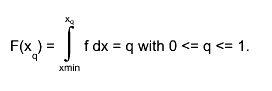$F(x_{q}) = #int_{xmin}^{x_{q}} f dx = q with 0 <= q <= 1.$
For instance the median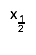$x_{#frac{1}{2}}$ of a distribution is defined as that value
of the random variable for which the distribution function equals 0.5: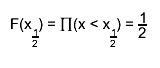$F(x_{#frac{1}{2}}) = #prod(x < x_{#frac{1}{2}}) = #frac{1}{2}$
code from Eddy Offermann, Renaissance

input parameters
- this TF1 function
- nprobSum maximum size of array q and size of array probSum
- probSum array of positions where quantiles will be computed.
It is assumed to contain at least nprobSum values.
output
- return value nq (<=nprobSum) with the number of quantiles computed
- array q filled with nq quantiles

Getting quantiles from two histograms and storing results in a TGraph,
a so-called QQ-plot

TGraph *gr = new TGraph(nprob);
f1->GetQuantiles(nprob,gr->GetX());
f2->GetQuantiles(nprob,gr->GetY());
gr->Draw("alp");
```

``` Return a random number following this function shape

The distribution contained in the function fname (TF1) is integrated
over the channel contents.
It is normalized to 1.
For each bin the integral is approximated by a parabola.
The parabola coefficients are stored as non persistent data members
Getting one random number implies:
- Generating a random number between 0 and 1 (say r1)
- Look in which bin in the normalized integral r1 corresponds to
- Evaluate the parabolic curve in the selected bin to find
the corresponding X value.
The parabolic approximation is very good as soon as the number
of bins is greater than 50.
```
Double_t GetRandom(Double_t xmin, Double_t xmax)
``` Return a random number following this function shape in [xmin,xmax]

The distribution contained in the function fname (TF1) is integrated
over the channel contents.
It is normalized to 1.
For each bin the integral is approximated by a parabola.
The parabola coefficients are stored as non persistent data members
Getting one random number implies:
- Generating a random number between 0 and 1 (say r1)
- Look in which bin in the normalized integral r1 corresponds to
- Evaluate the parabolic curve in the selected bin to find
the corresponding X value.
The parabolic approximation is very good as soon as the number
of bins is greater than 50.

IMPORTANT NOTE
The integral of the function is computed at fNpx points. If the function
has sharp peaks, you should increase the number of points (SetNpx)
such that the peak is correctly tabulated at several points.
```
void GetRange(Double_t& xmin, Double_t& xmax) const
``` Return range of a 1-D function.
```
void GetRange(Double_t& xmin, Double_t& ymin, Double_t& xmax, Double_t& ymax) const
``` Return range of a 2-D function.
```
void GetRange(Double_t& xmin, Double_t& ymin, Double_t& zmin, Double_t& xmax, Double_t& ymax, Double_t& zmax) const
``` Return range of function.
```
Double_t GetSave(const Double_t* x)
``` Get value corresponding to X in array of fSave values
```

``` Get x axis of the function.
```

``` Get y axis of the function.
```

``` Get z axis of the function. (In case this object is a TF2 or TF3)
```
Double_t GradientPar(Int_t ipar, const Double_t *x, Double_t eps)
``` Compute the gradient (derivative) wrt a parameter ipar
Parameters:
ipar - index of parameter for which the derivative is computed
x - point, where the derivative is computed
eps - if the errors of parameters have been computed, the step used in
numerical differentiation is eps*parameter_error.
if the errors have not been computed, step=eps is used
default value of eps = 0.01
Method is the same as in Derivative() function

If a paramter is fixed, the gradient on this parameter = 0
```
``` Compute the gradient wrt parameters
Parameters:
x - point, were the gradient is computed
grad - used to return the computed gradient, assumed to be of at least fNpar size
eps - if the errors of parameters have been computed, the step used in
numerical differentiation is eps*parameter_error.
if the errors have not been computed, step=eps is used
default value of eps = 0.01
Method is the same as in Derivative() function

If a paramter is fixed, the gradient on this parameter = 0
```
void InitArgs(const Double_t* x, const Double_t* params)
``` Initialize parameters addresses.
```
void InitStandardFunctions()
``` Create the basic function objects
```
Double_t Integral(Double_t a, Double_t b, const Double_t* params = 0, Double_t epsilon = 1e-12)
``` Return Integral of function between a and b.

based on original CERNLIB routine DGAUSS by Sigfried Kolbig
converted to C++ by Rene Brun

This function computes, to an attempted specified accuracy, the value
of the integral.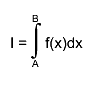$I = #int^{B}_{A} f(x)dx$
Usage:
In any arithmetic expression, this function has the approximate value
of the integral I.
- A, B: End-points of integration interval. Note that B may be less
than A.
- params: Array of function parameters. If 0, use current parameters.
- epsilon: Accuracy parameter (see Accuracy).

Method:
For any interval [a,b] we define g8(a,b) and g16(a,b) to be the 8-point
and 16-point Gaussian quadrature approximations to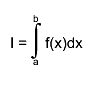$I = #int^{b}_{a} f(x)dx$
and define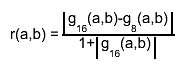$r(a,b) = #frac{#||{g_{16}(a,b)-g_{8}(a,b)}}{1+#||{g_{16}(a,b)}}$
Then,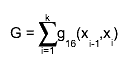$G = #sum_{i=1}^{k}g_{16}(x_{i-1},x_{i})$
where, starting with x0 = A and finishing with xk = B,
the subdivision points xi(i=1,2,...) are given by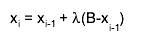$x_{i} = x_{i-1} + #lambda(B-x_{i-1})$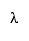$#lambda$ is equal to the first member of the
sequence 1,1/2,1/4,... for which r(xi-1, xi) < EPS.
If, at any stage in the process of subdivision, the ratio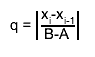$q = #||{#frac{x_{i}-x_{i-1}}{B-A}}$
is so small that 1+0.005q is indistinguishable from 1 to
machine accuracy, an error exit occurs with the function value
set equal to zero.

Accuracy:
Unless there is severe cancellation of positive and negative values of
f(x) over the interval [A,B], the argument EPS may be considered as
specifying a bound on the <I>relative</I> error of I in the case
|I|&gt;1, and a bound on the absolute error in the case |I|&lt;1. More
precisely, if k is the number of sub-intervals contributing to the
approximation (see Method), and if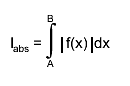$I_{abs} = #int^{B}_{A} #||{f(x)}dx$
then the relation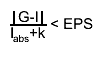$#frac{#||{G-I}}{I_{abs}+k} < EPS$
will nearly always be true, provided the routine terminates without
printing an error message. For functions f having no singularities in
the closed interval [A,B] the accuracy will usually be much higher than
this.

Error handling:
The requested accuracy cannot be obtained (see Method).
The function value is set equal to zero.

Note 1:
Values of the function f(x) at the interval end-points A and B are not
required. The subprogram may therefore be used when these values are
undefined.

Note 2:
Instead of TF1::Integral, you may want to use the combination of
TF1::CalcGaussLegendreSamplingPoints and TF1::IntegralFast.
See an example with the following script:

void gint() {
TF1 *g = new TF1("g","gaus",-5,5);
g->SetParameters(1,0,1);
//default gaus integration method uses 6 points
//not suitable to integrate on a large domain
double r1 = g->Integral(0,5);
double r2 = g->Integral(0,1000);

//try with user directives computing more points
Int_t np = 1000;
double *x=new double[np];
double *w=new double[np];
g->CalcGaussLegendreSamplingPoints(np,x,w,1e-15);
double r3 = g->IntegralFast(np,x,w,0,5);
double r4 = g->IntegralFast(np,x,w,0,1000);
double r5 = g->IntegralFast(np,x,w,0,10000);
double r6 = g->IntegralFast(np,x,w,0,100000);
printf("g->Integral(0,5)               = %g\n",r1);
printf("g->Integral(0,1000)            = %g\n",r2);
printf("g->IntegralFast(n,x,w,0,5)     = %g\n",r3);
printf("g->IntegralFast(n,x,w,0,1000)  = %g\n",r4);
printf("g->IntegralFast(n,x,w,0,10000) = %g\n",r5);
printf("g->IntegralFast(n,x,w,0,100000)= %g\n",r6);
delete [] x;
delete [] w;
}

This example produces the following results:

g->Integral(0,5)               = 1.25331
g->Integral(0,1000)            = 1.25319
g->IntegralFast(n,x,w,0,5)     = 1.25331
g->IntegralFast(n,x,w,0,1000)  = 1.25331
g->IntegralFast(n,x,w,0,10000) = 1.25331
g->IntegralFast(n,x,w,0,100000)= 1.253
```
Double_t Integral(Double_t ax, Double_t bx, Double_t ay, Double_t by, Double_t epsilon = 1e-12)
``` Return Integral of a 2d function in range [ax,bx],[ay,by]
```
Double_t Integral(Double_t ax, Double_t bx, Double_t ay, Double_t by, Double_t az, Double_t bz, Double_t epsilon = 1e-12)
``` Return Integral of a 3d function in range [ax,bx],[ay,by],[az,bz]
```
Double_t IntegralError(Double_t a, Double_t b, Double_t epsilon = 1e-12)
``` Return Error on Integral of a parameteric function between a and b due to the parameters uncertainties
It is assumed the parameters are estimated from a fit and the covariance matrix resulting from the fit is used in
estimating this error.
IMPORTANT NOTE: The calculation is valid assuming the parameters are resulting from the latest fit. If in the meantime
a fit is done using another function, the routine will signal  an error and return zero.
```
Double_t IntegralFast(const TGraph *g, Double_t a, Double_t b, Double_t *params)
``` Gauss-Legendre integral, see CalcGaussLegendreSamplingPoints
```
Double_t IntegralMultiple(Int_t n, const Double_t* a, const Double_t* b, Double_t epsilon, Double_t& relerr)
```  See more general prototype below.
This interface kept for back compatibility
```
Double_t IntegralMultiple(Int_t n, const Double_t* a, const Double_t* b, Int_t minpts, Int_t maxpts, Double_t epsilon, Double_t& relerr, Int_t& nfnevl, Int_t& ifail)
```  Adaptive Quadrature for Multiple Integrals over N-Dimensional
Rectangular Regions$I_{n} = #int_{a_{n}}^{b_{n}} #int_{a_{n-1}}^{b_{n-1}} ... #int_{a_{1}}^{b_{1}} f(x_{1}, x_{2},...,x_{n}) dx_{1}dx_{2}...dx_{n}$

Author(s): A.C. Genz, A.A. Malik
The new code features many changes compared to the Fortran version.
Note that this function is currently called only by TF2::Integral (n=2)
and TF3::Integral (n=3).

This function computes, to an attempted specified accuracy, the value of
the integral over an n-dimensional rectangular region.

Input parameters:

n     : Number of dimensions [2,15]
a,b   : One-dimensional arrays of length >= N . On entry A[i],  and  B[i],
contain the lower and upper limits of integration, respectively.
minpts: Minimum number of function evaluations requested. Must not exceed maxpts.
if minpts < 1 minpts is set to 2^n +2*n*(n+1) +1
maxpts: Maximum number of function evaluations to be allowed.
maxpts >= 2^n +2*n*(n+1) +1
if maxpts<minpts, maxpts is set to 10*minpts
eps   : Specified relative accuracy.

Output parameters:

relerr : Contains, on exit, an estimation of the relative accuracy of the result.
nfnevl : number of function evaluations performed.
ifail  :
0 Normal exit.  . At least minpts and at most maxpts calls to the function were performed.
1 maxpts is too small for the specified accuracy eps.
The result and relerr contain the values obtainable for the
specified value of maxpts.
3 n<2 or n>15

Method:

An integration rule of degree seven is used together with a certain
strategy of subdivision.
For a more detailed description of the method see References.

Notes:

1.Multi-dimensional integration is time-consuming. For each rectangular
subregion, the routine requires function evaluations.
Careful programming of the integrand might result in substantial saving
of time.
2.Numerical integration usually works best for smooth functions.
Some analysis or suitable transformations of the integral prior to
numerical work may contribute to numerical efficiency.

References:

1.A.C. Genz and A.A. Malik, Remarks on algorithm 006:
An adaptive algorithm for numerical integration over
an N-dimensional rectangular region, J. Comput. Appl. Math. 6 (1980) 295-302.
2.A. van Doren and L. de Ridder, An adaptive algorithm for numerical
integration over an n-dimensional cube, J.Comput. Appl. Math. 2 (1976) 207-217.
```
Bool_t IsInside(const Double_t* x) const
``` Return kTRUE is the point is inside the function range
```
void Paint(Option_t* option = "")
``` Paint this function with its current attributes.
```
void Print(Option_t* option = "") const
``` Dump this function with its attributes.
```
void ReleaseParameter(Int_t ipar)
``` Release parameter number ipar If used in a fit, the parameter
can vary freely. The parameter limits are reset to 0,0.
```
void Save(Double_t xmin, Double_t xmax, Double_t ymin, Double_t ymax, Double_t zmin, Double_t zmax)
``` Save values of function in array fSave
```
void SavePrimitive(ostream& out, Option_t* option = "")
``` Save primitive as a C++ statement(s) on output stream out
```
void SetCurrent(TF1* f1)
``` Static function setting the current function.
the current function may be accessed in static C-like functions
when fitting or painting a function.
```
void SetMaximum(Double_t maximum = -1111)
``` Set the maximum value along Y for this function
In case the function is already drawn, set also the maximum in the
helper histogram
```
void SetMinimum(Double_t minimum = -1111)
``` Set the minimum value along Y for this function
In case the function is already drawn, set also the minimum in the
helper histogram
```
void SetNDF(Int_t ndf)
``` Set the number of degrees of freedom
ndf should be the number of points used in a fit - the number of free parameters
```
void SetNpx(Int_t npx = 100)
``` Set the number of points used to draw the function

The default number of points along x is 100 for 1-d functions and 30 for 2-d/3-d functions
You can increase this value to get a better resolution when drawing
pictures with sharp peaks or to get a better result when using TF1::GetRandom
the minimum number of points is 4, the maximum is 100000 for 1-d and 10000 for 2-d/3-d functions
```
void SetParError(Int_t ipar, Double_t error)
``` Set error for parameter number ipar
```
void SetParErrors(const Double_t* errors)
``` Set errors for all active parameters
when calling this function, the array errors must have at least fNpar values
```
void SetParLimits(Int_t ipar, Double_t parmin, Double_t parmax)
``` Set limits for parameter ipar.

The specified limits will be used in a fit operation
when the option "B" is specified (Bounds).
To fix a parameter, use TF1::FixParameter
```
void SetRange(Double_t xmin, Double_t xmax)
``` Initialize the upper and lower bounds to draw the function.

The function range is also used in an histogram fit operation
when the option "R" is specified.
```
void SetSavedPoint(Int_t point, Double_t value)
``` Restore value of function saved at point
```
void SetTitle(const char* title = "")
``` Set function title
if title has the form "fffffff;xxxx;yyyy", it is assumed that
the function title is "fffffff" and "xxxx" and "yyyy" are the
titles for the X and Y axis respectively.
```
void Streamer(TBuffer& b)
``` Stream a class object.
```
void Update()
``` Called by functions such as SetRange, SetNpx, SetParameters
to force the deletion of the associated histogram or Integral
```
void RejectPoint(Bool_t reject = kTRUE)
``` Static function to set the global flag to reject points
the fgRejectPoint global flag is tested by all fit functions
if TRUE the point is not included in the fit.
This flag can be set by a user in a fitting function.
The fgRejectPoint flag is reset by the TH1 and TGraph fitting functions.
```

``` See TF1::RejectPoint above
```
Double_t Moment(Double_t n, Double_t a, Double_t b, const Double_t* params = 0, Double_t epsilon = 0.000001)
``` Return nth moment of function between a and b

See TF1::Integral() for parameter definitions
Author: Gene Van Buren <gene@bnl.gov>
```
Double_t CentralMoment(Double_t n, Double_t a, Double_t b, const Double_t* params = 0, Double_t epsilon = 0.000001)
``` Return nth central moment of function between a and b

See TF1::Integral() for parameter definitions
Author: Gene Van Buren <gene@bnl.gov>
```
void CalcGaussLegendreSamplingPoints(TGraph *g, Double_t eps)
``` Type safe interface (static method)
The number of sampling points are taken from the TGraph
```
void SetRange(Double_t xmin, Double_t ymin, Double_t xmax, Double_t ymax)
`{ TF1::SetRange(xmin, xmax); }`
void SetRange(Double_t xmin, Double_t ymin, Double_t zmin, Double_t xmax, Double_t ymax, Double_t zmax)
`{ TF1::SetRange(xmin, xmax); }`
Double_t operator()(const Double_t* x, const Double_t* params = 0)
``` for using TF1 as a callable object (functor)
```
`{ return EvalPar(x,params); }`

`{return fChisquare;}`

`{return fNpx;}`

`{return fMethodCall;}`

`{return fNpfits;}`

`{return fParent;}`

`{return fParErrors;}`

`{return fXmin;}`

`{return fXmax;}`
void SetChisquare(Double_t chi2)
`{fChisquare = chi2;}`
void SetFunction(Double_t (*fcn)(Double_t *, Double_t *))
`{ fFunctor = ROOT::Math::ParamFunctor(fcn);}`
void SetNumberFitPoints(Int_t npfits)
`{fNpfits = npfits;}`
void SetParent(TObject* p = 0)
`{fParent = p;}`
Double_t Mean(Double_t a, Double_t b, const Double_t* params = 0, Double_t epsilon = 0.000001)
`{return Moment(1,a,b,params,epsilon);}`
Double_t Variance(Double_t a, Double_t b, const Double_t* params = 0, Double_t epsilon = 0.000001)
`{return CentralMoment(2,a,b,params,epsilon);}`

Author: Rene Brun 18/08/95
Last update: root/hist:\$Id: TF1.h 20882 2007-11-19 11:31:26Z rdm \$